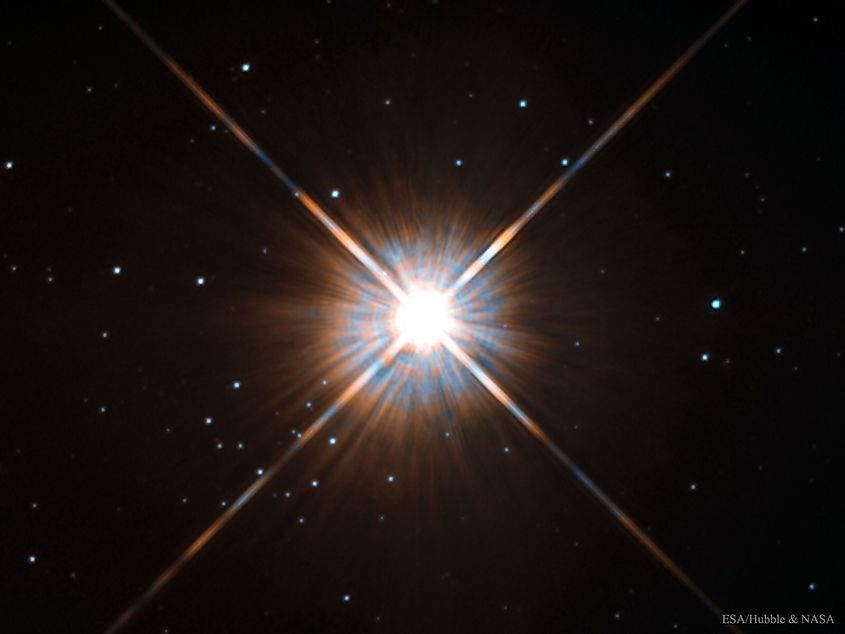# What Are Parsecs?

Objects in space are located at tremendous distances from our solar system. Everyday units such as miles or kilometres simply become too large and complicated to use when objects are trillions upon trillions of miles away. To simplify things, astronomers generally use light years, which is just the distance light travels over the course of a single year. For example, a star that is located 500 light years away would take 500 years to reach travelling at the speed of light. There is actually another form of measurement that is often used in calculating vast distances: parsecs.

## How Big Are Parsecs?Proxima Centauri is the closest star to the sun at a distance of 4.2 light years or 1.3 parsecs. Image credit: NASA/ESA

One parsec is equivalent to 3.26 light years. The nearest star to us, Proxima Centauri, is located 4.2 light years away or 1.3 parsecs. Going from light year to parsec is fairly simple, and all it requires is dividing the number of light years by 3.26. However, why is one parsec equal to 3.26 light years? After all, that number seems fairly specific. Parsecs are calculated using parallax, which is a form of distance measurement used in astronomical observations. Using parallax, astronomers calculate the distance it takes for one astronomical unit to subtend one arcsecond. One astronomical unit is equal to the distance between the Earth and sun, while arcseconds are a unit for measuring angles (one arcsecond is equal to 1/3600 degrees). So a parsec is the calculated distance it takes for one astronomical unit of distance to cover an angle of 1/3600 degrees. This distance is 3.26 light years or one parsec.

## Larger Units Of ParsecAstronomers will generally use megaparsecs or gigaparsecs to measure the distances to the furthest galaxies. Image credit: NASA/ESA

To simplify even larger distances, astronomers also use kiloparsecs, megaparsecs, and gigaparsecs. A kiloparsec is equal to 1,000 parsecs, and it is generally used in measuring distances to objects in and around the Milky Way. Megaparsecs are equal to one-million parsecs, and are used in measuring distances to other galaxies. The largest unit, gigaparsecs, are equal to one-billion parsecs, and thus they are used in measuring the furthest distances in space.

## Distance Units In Astronomy

Unit Amount/Definition

Astronomical Unit

93-million miles (150-million kilometres), or the distance between Earth and the sun

Light Year

The distance light travels in one year, equal to 5.88 trillion miles (9.46 trillion kilometres)

Parsec

Distance it takes one astronomical unit to cover an angle of one arcsecond, equal to 3.26 light years

Kiloparsec

1,000 parsecs

Megaparsec

One-million parsecs

Gigaparsec

One-billion parsecs

Share